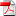# Variance optimal hedging with application to Electricity markets - Xavier Warin

11
Déc

In this article, we use the mean variance hedging criterion to value contracts in incomplete markets. Although the problem is well studied in a continuous and even discrete framework, very few works incorporating illiquidity constraints have been achieved and no algorithm is available in the literature to solve this problem. We ﬁrst show that the valuation problem incorporating illiquidity constraints with a mean variance criterion admits a unique solution. Then we develop two Least Squares Monte Carlo algorithms based on the dynamic programming principle to eﬃciently value these contracts: in these methods, conditional expectations are classically calculated by regression using a dynamic programming approach discretizing the control. The ﬁrst algorithm calculates the optimal value function while the second calculates the optimal cash ﬂow generated along the trajectories. In a third part, we give the example of the valuation of a load curve contract coming from energy markets. In such contracts, incompleteness comes from the uncertainty of the customer’s load that cannot be hedged and very tight illiquity constraints are present. We compare strategies given by the two algorithms and by some closed formula ignoring constraints and we show that hedging strategies can be very diﬀerent. A numerical study of the convergence of the algorithms is also given.

•RR-FIME-18-06